Before discussing the Prim’s Algorithm let’s have a brief   introduction of the Graph Theory, Graph theory discusses about the relationship between lines and points. It is an important branch in the Mathematics and in Computer Science. Mathematician Euler is considered to be Father of Graph theory as he researched a lot in Graph Theory and gave many definitions and type of graphs , one of the graph in his respect is termed as  Euler Graph .  Here we will discuss Algorithms for simplification of the Graphs.

Prim’s Algorithm is also known as greedy algorithm, it is an important branch in Mathematics. It also creates spanning tree. A spanning Tree is a graph in which all the nodes are connected. The objective of Prim’s algorithm is creating Spanning tree. This algorithm also works on idea how a spanning tree grow. This algorithm is called greedy algorithm because it selects  the next node depending upon the best approach not on straight forward rule.  It provides the flexibility to select any edge to make light cut at each iteration. A cut is selecting next best node depending upon minimum weight and connecting the previous node from this node.

## Method of Implementation of The Prim’s Algorithm

• Observe all the edges and their weight.
• Observe those edges who are not connected.
• Assign a key value to each node, depending upon the order of the node.
• Now select the node that is not connected (term it as root node-r) and has minimum weight and include it in connected edges or in minimum spanning tree.
• Now select the next nearest node with minimum weight and include that node in the minimum spanning tree.
• Repeat the same procedure until all the nodes are not connected.

## Kruskal’s Algorithm and Prim’s Algorithm

Both Kruskal and Prim Algorithm are greedy algorithm and take cut with minimum weight. The major difference between both the algorithms is that kruskal’s Algorithm concentrates on edge and Prim’s Algorithm is Node.

Practical ExampleStep1 – Select Node- a as    the   root node, observe the weight on adjacent nodes –b and c, here on comparison the weight of edge a to b is minimum so selecting this cut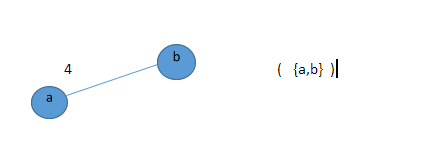Step2 – From   Node   b the adjacent nodes are c,  d and e, the minimum weight is on the   edge from b to d, select cut from b to d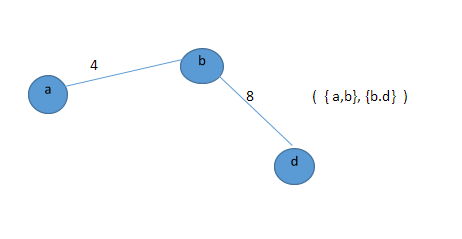Step3 – From Node d the adjacent nodes are c, f  and e, by observing the minimum weight   is on edge from d to c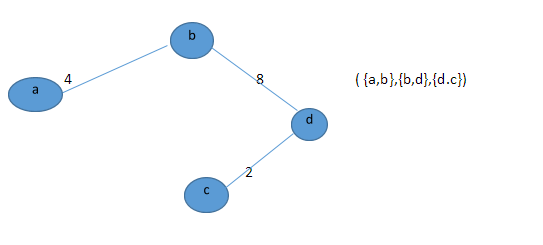Step 4 – From node C the adjacent nodes are a and f, now the weight of edge from node  c to f has to be selected as node a has already covered –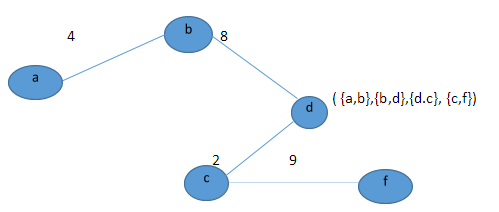Step 5 – From node f the adjacent nodes are d, e and g, as d has already covered so it cannot be selected , now observing nodes e and g , the weight from f to g is minimum.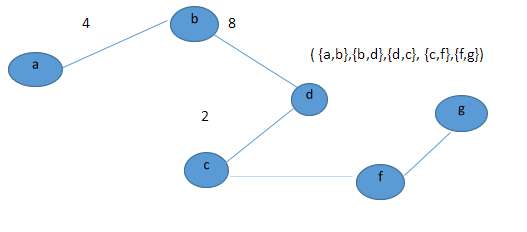Step 6 – From node g the adjacent node are f and e, f is already covered so e has to be selected### Importance of The Prim’s Algorithm

The Prim’s Algorithm is very simple and easy to implement, it helps to simplify the graph in easy way. It is simple and straight forward and easy to work on it.

Author:
Category :
Publish Date :
Social Sharing